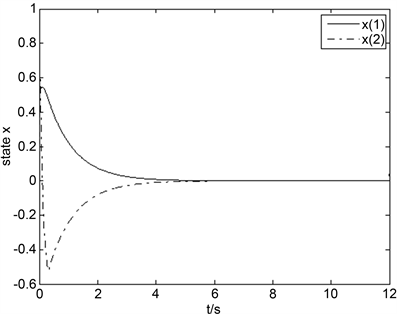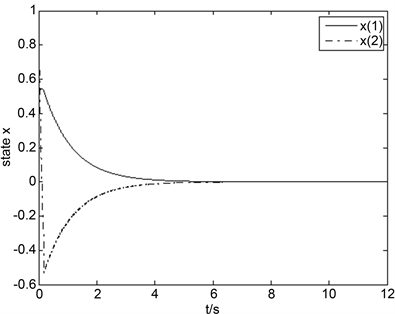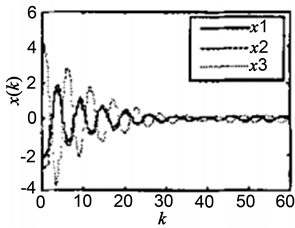# 不确定性T-S模糊时滞系统的容错控制研究Fault-Tolerant Control for Uncertain T-S Fuzzy Time-Delay Systems

DOI: 10.12677/AAM.2020.91014, PDF, HTML, XML, 下载: 162  浏览: 250

Abstract: This paper is concerned with fault-tolerant control for a class of nonlinear continuous systems with uncertainties and delay terms. Firstly, the T-S fuzzy model is constructed to approximate the original system more accurately, and the Lyapunov function is constructed, the robustness of the closed-loop system with actuator faults is guaranteed by the designed fuzzy controller. The value of gain matrix and the feasible conditions for the closed-loop system are proposed by solving linear matrix inequalities. Finally, the effectiveness of the method is verified by numerical simulation.

1. 引言

T-S模糊模型是一种用来描述复杂系统，有效解决线性与非线性控制系统之间差距的模糊模型，即用模糊规则来描述非线性系统，进而达到对非线性系统建模的目的。利用T-S模糊控制方法来解决系统中的非线性和不确定问题也得到了广泛的关注和研究，并取得了一系列成果。

2. 系统描述

$\left\{\begin{array}{l}\stackrel{˙}{x}\left(t\right)=\left({A}_{i}+\Delta {A}_{i}\right)x\left(t\right)+\left({A}_{di}+\Delta {A}_{di}\right)x\left(t-d\right)+\left({B}_{i}+\Delta {B}_{i}\right)u\left(t\right)+w\left(t\right),\\ z\left(t\right)={C}_{i}x\left(t\right),\\ x\left(t\right)=\phi \left(t\right),t\in \left[-d,0\right],i=1,2,\cdots ,r.\end{array}$ (1)

$\left[\Delta {A}_{i}\left(t\right),\Delta {B}_{i}\left(t\right),\Delta {A}_{di}\left(t\right)\right]=M{F}_{i}\left(t\right)\left[{E}_{1i},{E}_{2i},{E}_{di}\right]$(2)

${F}_{i}\left(t\right){F}_{i}^{\text{T}}\left(t\right)\le I$(3)

$\left\{\begin{array}{l}\stackrel{˙}{x}\left(t\right)={\sum }_{i=1}^{r}{b}_{i}\left(\theta \left(t\right)\right)\left[\left({A}_{i}+\Delta {A}_{i}\right)x\left(t\right)+\left({A}_{di}+\Delta {A}_{di}\right)x\left(t-d\right)+\left({B}_{i}+\Delta {B}_{i}\right)u\left(t\right)+w\left(t\right)\right]\\ z\left(t\right)={\sum }_{i=1}^{r}{b}_{i}\left(\theta \left(t\right)\right){C}_{i}x\left(t\right)\end{array}$ (4)

$u\left(t\right)={\sum }_{i=1}^{r}{b}_{i}\left(\theta \left(t\right)\right){K}_{i}x\left(t\right),i=1,2,\cdots ,r$ (5)

$\begin{array}{c}\stackrel{˙}{x}\left(t\right)={\sum }_{i=1}^{r}{\sum }_{j=1}^{r}{b}_{i}\left(\theta \left(t\right)\right){b}_{j}\left(\theta \left(t\right)\right)\left[\left({A}_{i}+\Delta {A}_{i}\left(t\right)+{B}_{i}{K}_{j}+\Delta {B}_{i}\left(t\right){K}_{j}\right)x\left(t\right)\\ \text{\hspace{0.17em}}\text{\hspace{0.17em}}+\left({A}_{di}+\Delta {A}_{di}\left(t\right)\right)x\left(t-d\right)+w\left(t\right)\right]\end{array}$ (6)

$L=diag\left({l}_{1},{l}_{2},\cdots ,{l}_{n}\right)$

${l}_{i}=\left\{\begin{array}{l}0,第i个执行器失效\\ 1,第i个执行器正常\end{array}$

$\begin{array}{c}\stackrel{˙}{x}\left(t\right)={\sum }_{i=1}^{r}{\sum }_{j=1}^{r}{b}_{i}\left(\theta \left(t\right)\right){b}_{j}\left(\theta \left(t\right)\right)\left[\left({A}_{i}+\Delta {A}_{i}\left(t\right)+{B}_{i}L{K}_{j}+\Delta {B}_{i}\left(t\right)L{K}_{j}\right)x\left(t\right)\\ \text{\hspace{0.17em}}\text{\hspace{0.17em}}+\left({A}_{di}+\Delta {A}_{di}\left(t\right)\right)x\left(t-d\right)+w\left(t\right)\right]\end{array}$ (7)

① 当干扰 $w\left(t\right)=0$ 时，证明闭环系统(7)是渐进稳定的；

② 当干扰 $w\left(t\right)\ne 0$ 时，证明对任意干扰衰减指标 $\gamma$ ，若系统控制输出 $z\left(t\right)$ 满足 ${‖z\left(t\right)‖}_{2}\le \gamma {‖w\left(t\right)‖}_{2}$ ，闭环系统(7)是渐进稳定的。

3. 主要结果

${x}_{1}^{\text{T}}Y{x}_{2}+{x}_{2}^{\text{T}}{Y}^{\text{T}}{x}_{1}\le {x}_{1}^{\text{T}}Y{R}^{-1}{Y}^{\text{T}}{x}_{1}+{x}_{2}^{\text{T}}R{x}_{2}$

$S<0$

${S}_{11}<0,{S}_{22}-{S}_{12}^{\text{T}}{S}_{11}^{-1}{S}_{12}<0$

${S}_{22}<0,{S}_{11}-{S}_{12}{S}_{22}^{-1}{S}_{12}^{\text{T}}<0$

$\left[\begin{array}{ccccc}\Psi & XR& {A}_{di}& X{E}_{1i}^{\text{T}}+{Y}_{j}^{\text{T}}L{E}_{2i}^{\text{T}}& M\\ *& -R& 0& 0& 0\\ *& *& -R& {E}_{di}^{\text{T}}& 0\\ *& *& *& -\alpha I& 0\\ *& *& *& *& -{\alpha }^{-1}I\end{array}\right]<0$ (8)

$\Psi =X{A}_{i}^{\text{T}}+{A}_{i}X+{Y}_{j}^{\text{T}}L{B}_{i}^{\text{T}}+{B}_{i}L{Y}_{j}$

$V\left(x\left(t\right)\right)={x}^{\text{T}}\left(t\right)Px\left(t\right)+\underset{t-d}{\overset{t}{\int }}{x}^{\text{T}}\left(s\right)Rx\left(s\right)\text{d}s$

$\begin{array}{c}\stackrel{˙}{V}\left(x\left(t\right)\right)={\stackrel{˙}{x}}^{\text{T}}\left(t\right)px\left(t\right)+{x}^{\text{T}}\left(t\right)p\stackrel{˙}{x}\left(t\right)+{x}^{\text{T}}\left(t\right)Rx\left(t\right)-{x}^{\text{T}}\left(t-d\right)Rx\left(t-d\right)\\ =\underset{i=1}{\overset{r}{\sum }}\underset{j=1}{\overset{r}{\sum }}{b}_{i}\left(\theta \left(t\right)\right){b}_{j}\left(\theta \left(t\right)\right)\left[{x}^{\text{T}}\left(t\right){\left({A}_{i}+{B}_{i}L{K}_{j}+\Delta {A}_{i}\left(t\right)+\Delta {B}_{i}\left(t\right)L{K}_{j}\right)}^{\text{T}}Px\left(t\right)\\ \text{\hspace{0.17em}}\text{\hspace{0.17em}}+{x}^{\text{T}}\left(t-d\right){\left({A}_{di}+\Delta {A}_{di}\left(t\right)\right)}^{\text{T}}Px\left(t\right)+{x}^{\text{T}}\left(t\right)P\left({A}_{i}+{B}_{i}L{K}_{j}+\Delta {A}_{i}\left(t\right)+\Delta {B}_{i}\left(t\right)L{K}_{j}\right)x\left(t\right)\\ \text{\hspace{0.17em}}\text{\hspace{0.17em}}+{x}^{\text{T}}\left(t\right)P\left({A}_{di}+\Delta {A}_{di}\left(t\right)\right)x\left(t-d\right)+{x}^{\text{T}}\left(t\right)Rx\left(t\right)-{x}^{\text{T}}\left(t-d\right)Rx\left(t-d\right)\right]\end{array}$

$\begin{array}{l}\le \underset{i=1}{\overset{r}{\sum }}\underset{j=1}{\overset{r}{\sum }}{b}_{i}\left(\theta \left(t\right)\right){b}_{j}\left(\theta \left(t\right)\right)\left[{x}^{\text{T}}\left(t\right)\left({\left({A}_{i}+{B}_{i}L{K}_{j}+\Delta {A}_{i}\left(t\right)+\Delta {B}_{i}\left(t\right)L{K}_{j}\right)}^{\text{T}}P\\ \text{\hspace{0.17em}}\text{\hspace{0.17em}}\text{ }+P\left({A}_{i}+{B}_{i}L{K}_{j}+\Delta {A}_{i}\left(t\right)+\Delta {B}_{i}\left(t\right)L{K}_{j}\right)\right)x\left(t\right)\\ \text{\hspace{0.17em}}\text{\hspace{0.17em}}\text{ }+{x}^{\text{T}}\left(t\right)P\left({A}_{di}+\Delta {A}_{di}\left(t\right)\right){R}^{-1}{\left({A}_{di}+\Delta {A}_{di}\left(t\right)\right)}^{\text{T}}Px\left(t\right)\\ \text{\hspace{0.17em}}\text{\hspace{0.17em}}\text{ }+{x}^{\text{T}}\left(t-d\right)Rx\left(t-d\right)+{x}^{\text{T}}\left(t\right)Rx\left(t\right)-{x}^{\text{T}}\left(t-d\right)Rx\left(t-d\right)\right]\end{array}$

$\begin{array}{l}=\underset{i=1}{\overset{r}{\sum }}\underset{j=1}{\overset{r}{\sum }}{b}_{i}\left(\theta \left(t\right)\right){b}_{j}\left(\theta \left(t\right)\right)\left[{x}^{\text{T}}\left(t\right)\left({\left({A}_{i}+{B}_{i}L{K}_{j}+\Delta {A}_{i}\left(t\right)+\Delta {B}_{i}\left(t\right)L{K}_{j}\right)}^{\text{T}}P\\ \text{\hspace{0.17em}}\text{\hspace{0.17em}}\text{ }\text{ }+P\left({A}_{i}+{B}_{i}L{K}_{j}+\Delta {A}_{i}\left(t\right)+\Delta {B}_{i}\left(t\right)L{K}_{j}\right)\right)x\left(t\right)\\ \text{\hspace{0.17em}}\text{\hspace{0.17em}}\text{ }\text{ }+{x}^{\text{T}}\left(t\right)P\left({A}_{di}+\Delta {A}_{di}\left(t\right)\right){R}^{-1}{\left({A}_{di}+\Delta {A}_{di}\left(t\right)\right)}^{\text{T}}Px\left(t\right)+{x}^{\text{T}}\left(t\right)Rx\left(t\right)\right]\end{array}$

$\begin{array}{l}=\underset{i=1}{\overset{r}{\sum }}\underset{j=1}{\overset{r}{\sum }}{b}_{i}\left(\theta \left(t\right)\right){b}_{j}\left(\theta \left(t\right)\right)\left[{x}^{\text{T}}\left(t\right)\left({\left({A}_{i}+{B}_{i}L{K}_{j}+\Delta {A}_{i}\left(t\right)+\Delta {B}_{i}\left(t\right)L{K}_{j}\right)}^{\text{T}}P\\ \text{\hspace{0.17em}}\text{\hspace{0.17em}}\text{ }\text{ }+P\left({A}_{i}+{B}_{i}L{K}_{j}+\Delta {A}_{i}\left(t\right)+\Delta {B}_{i}\left(t\right)L{K}_{j}\right)\\ \text{\hspace{0.17em}}\text{\hspace{0.17em}}\text{ }\text{ }+R+P\left({A}_{di}+\Delta {A}_{di}\left(t\right)\right){R}^{-1}{\left({A}_{di}+\Delta {A}_{di}\left(t\right)\right)}^{\text{T}}P\right)x\left(t\right)\right]\end{array}$

$\begin{array}{l}\left({\left({A}_{i}+{B}_{i}L{K}_{j}+\Delta {A}_{i}\left(t\right)+\Delta {B}_{i}\left(t\right)L{K}_{j}\right)}^{\text{T}}P\\ +P\left({A}_{i}+{B}_{i}L{K}_{j}+\Delta {A}_{i}\left(t\right)+\Delta {B}_{i}\left(t\right)L{K}_{j}\right)\\ \text{ }+R+P\left({A}_{di}+\Delta {A}_{di}\left(t\right)\right){R}^{-1}{\left({A}_{di}+\Delta {A}_{di}\left(t\right)\right)}^{\text{T}}P\right)<0\end{array}$

${\prod }_{1}={\left({A}_{i}+{B}_{i}L{K}_{j}+\Delta {A}_{i}\left(t\right)+\Delta {B}_{i}\left(t\right)L{K}_{j}\right)}^{\text{T}}P+P\left({A}_{i}+{B}_{i}L{K}_{j}+\Delta {A}_{i}\left(t\right)+\Delta {B}_{i}\left(t\right)L{K}_{j}\right)$

$\left[\Delta {A}_{i}\left(t\right),\Delta {B}_{i}\left(t\right),\Delta {A}_{di}\left(t\right)\right]=M{F}_{i}\left(t\right)\left[{E}_{1i},{E}_{2i},{E}_{di}\right]$ 带入(9)可得：

$\begin{array}{l}\left[\begin{array}{ccc}{\Pi }_{\text{1}}& R& P\left({A}_{di}+\Delta {A}_{di}\left(t\right)\right)\\ *& -R& 0\\ *& *& -R\end{array}\right]\\ =\left[\begin{array}{ccc}{\left({A}_{i}+{B}_{i}L{K}_{j}\right)}^{\text{T}}P+P\left({A}_{i}+{B}_{i}L{K}_{j}\right)& R& P{A}_{di}\\ *& -R& 0\\ *& *& -R\end{array}\right]\\ \text{\hspace{0.17em}}\text{\hspace{0.17em}}+\left[\begin{array}{c}PM\\ 0\\ 0\end{array}\right]{F}_{i}\left(t\right)\left[\begin{array}{ccc}{E}_{1i}+{E}_{2i}L{K}_{j}& 0& {E}_{di}\end{array}\right]\\ \text{\hspace{0.17em}}\text{\hspace{0.17em}}+\left[\begin{array}{c}{\left({E}_{1i}+{E}_{2i}L{K}_{j}\right)}^{\text{T}}\\ 0\\ {E}_{di}^{T}\end{array}\right]{F}_{i}^{\text{T}}\left(t\right)\left[\begin{array}{ccc}{M}^{\text{T}}P& 0& 0\end{array}\right]\end{array}$

$\begin{array}{l}\left[\begin{array}{ccc}{\left({A}_{i}+{B}_{i}L{K}_{j}\right)}^{\text{T}}P+P\left({A}_{i}+{B}_{i}L{K}_{j}\right)& R& P{A}_{di}\\ *& -R& 0\\ *& *& -R\end{array}\right]\\ \text{ }+\alpha \left[\begin{array}{c}PM\\ 0\\ 0\end{array}\right]\left[\begin{array}{ccc}{M}^{\text{T}}P& 0& 0\end{array}\right]+{\alpha }^{-1}\left[\begin{array}{c}{\left({E}_{1i}+{E}_{2i}L{K}_{j}\right)}^{\text{T}}\\ 0\\ {E}_{di}^{\text{T}}\end{array}\right]\left[\begin{array}{ccc}{E}_{1i}+{E}_{2i}L{K}_{j}& 0& {E}_{di}\end{array}\right]\\ =\left[\begin{array}{ccc}{\Pi }_{2}& R& P{A}_{di}+{\alpha }^{-1}{\left({E}_{1i}+{E}_{2i}L{K}_{j}\right)}^{\text{T}}{E}_{di}\\ *& -R& 0\\ *& *& -R+{\alpha }^{-1}{E}_{di}^{\text{T}}{E}_{di}\end{array}\right]<0\end{array}$ (10)

${\prod }_{2}={\left({A}_{i}+{B}_{i}L{K}_{j}\right)}^{\text{T}}P+P\left({A}_{i}+{B}_{i}L{K}_{j}\right)+\alpha PM{M}^{\text{T}}P+{\alpha }^{-1}{\left({E}_{1i}+{E}_{2i}L{K}_{j}\right)}^{\text{T}}\left({E}_{1i}+{E}_{2i}L{K}_{j}\right)$

$\left[\begin{array}{ccccc}{\prod }_{3}& R& P{A}_{di}& {\left({E}_{1i}+{E}_{2i}L{K}_{j}\right)}^{\text{T}}& PM\\ *& -R& 0& 0& 0\\ *& *& -R& {E}_{di}^{\text{T}}& 0\\ *& *& *& -\alpha I& 0\\ *& *& *& *& -{\alpha }^{-1}I\end{array}\right]<0$ (11)

${\prod }_{3}={\left({A}_{i}+{B}_{i}L{K}_{j}\right)}^{\text{T}}P+P\left({A}_{i}+{B}_{i}L{K}_{j}\right)$

$\left[\begin{array}{ccccc}\Psi & XR& {A}_{di}& X{E}_{1i}^{\text{T}}+{Y}_{j}^{\text{T}}L{E}_{2i}^{\text{T}}& M\\ *& -R& 0& 0& 0\\ *& *& -R& {E}_{di}^{\text{T}}& 0\\ *& *& *& -\alpha I& 0\\ *& *& *& *& -{\alpha }^{-1}I\end{array}\right]<0$

$\left[\begin{array}{ccccccc}\Psi & I& XR& {A}_{di}& X{C}_{i}& X{E}_{1i}+{Y}_{j}^{\text{T}}L{E}_{2i}& M\\ \text{*}& -{\gamma }^{2}I& 0& 0& 0& 0& 0\\ \text{*}& \text{*}& -R& 0& 0& 0& 0\\ \text{*}& \text{*}& \text{*}& -R& 0& {E}_{di}^{\text{T}}& 0\\ \text{*}& \text{*}& \text{*}& \text{*}& -I& 0& 0\\ \text{*}& \text{*}& \text{*}& \text{*}& \text{*}& -\alpha I& 0\\ \text{*}& \text{*}& \text{*}& \text{*}& \text{*}& \text{*}& -{\alpha }^{-1}I\end{array}\right]<0$ (12)

$\Psi =X{A}_{i}^{\text{T}}+{A}_{i}X+{Y}_{j}^{\text{T}}L{B}_{i}^{\text{T}}+{B}_{i}L{Y}_{j}$

$J={\int }_{0}^{\infty }\left[{z}^{\text{T}}\left(t\right)z\left(t\right)-{\gamma }^{2}{w}^{\text{T}}\left(t\right)w\left(t\right)\right]\text{d}t$ (13)

$\begin{array}{c}J={\int }_{0}^{\infty }\left[{z}^{\text{T}}\left(t\right)z\left(t\right)-{\gamma }^{2}{w}^{\text{T}}\left(t\right)w\left(t\right)\right]\text{d}t\\ ={\int }_{0}^{\infty }\left[{z}^{\text{T}}\left(t\right)z\left(t\right)-{\gamma }^{2}{w}^{\text{T}}\left(t\right)w\left(t\right)+\frac{\text{d}}{\text{d}t}V\left(x\left(t\right)\right)\right]\text{d}t-V\left(x\left(t\right)\right)\\ \le {\int }_{0}^{\infty }\left[{z}^{\text{T}}\left(t\right)z\left(t\right)-{\gamma }^{2}{w}^{\text{T}}\left(t\right)w\left(t\right)+\frac{\text{d}}{\text{d}t}V\left(x\left(t\right)\right)\right]\text{d}t\end{array}$ (14)

$\begin{array}{l}{z}^{\text{T}}\left(t\right)z\left(t\right)-{\gamma }^{2}{w}^{\text{T}}\left(t\right)w\left(t\right)+\frac{\text{d}}{\text{d}t}V\left(x\left(t\right)\right)\\ =\underset{i=1}{\overset{r}{\sum }}\underset{j=1}{\overset{r}{\sum }}{b}_{i}\left(\theta \left(t\right)\right){b}_{j}\left(\theta \left(t\right)\right)\left[{x}^{\text{T}}\left(t\right){C}_{i}^{\text{T}}{C}_{i}x\left(t\right)-{\gamma }^{2}{w}^{\text{T}}\left(t\right)w\left(t\right)+{x}^{\text{T}}\left(t\right)\left({A}_{i}+{B}_{i}L{K}_{j}\\ \text{\hspace{0.17em}}\text{\hspace{0.17em}}\text{ }\text{ }+\Delta {A}_{i}\left(t\right)+{\Delta {B}_{i}\left(t\right)L{K}_{j}\right)}^{\text{T}}Px\left(t\right)+{x}^{\text{T}}\left(t-d\right){\left({A}_{di}+\Delta {A}_{di}\left(t\right)\right)}^{\text{T}}Px\left(t\right)\\ \text{\hspace{0.17em}}\text{\hspace{0.17em}}\text{ }\text{ }+{x}^{\text{T}}\left(t\right)P\left({A}_{i}+{B}_{i}L{K}_{j}+\Delta {A}_{i}\left(t\right)+\Delta {B}_{i}\left(t\right)L{K}_{j}\right)x\left(t\right)+{w}^{\text{T}}\left(t\right)Px\left(t\right)+{x}^{\text{T}}\left(t\right)Pw\left(t\right)\\ \text{\hspace{0.17em}}\text{\hspace{0.17em}}\text{ }\text{ }+{x}^{\text{T}}\left(t\right)P\left({A}_{di}+\Delta {A}_{di}\left(t\right)\right)x\left(t-d\right)+{x}^{\text{T}}\left(t\right)Rx\left(t\right)-{x}^{\text{T}}\left(t-d\right)Rx\left(t-d\right)\right]\end{array}$

$\begin{array}{l}=\underset{i=1}{\overset{r}{\sum }}\underset{j=1}{\overset{r}{\sum }}{b}_{i}\left(\theta \left(t\right)\right){b}_{j}\left(\theta \left(t\right)\right)\left[{x}^{\text{T}}\left(t\right)\left({\left({A}_{i}+{B}_{i}L{K}_{j}+\Delta {A}_{i}\left(t\right)+\Delta {B}_{i}\left(t\right)L{K}_{j}\right)}^{\text{T}}P\\ \text{\hspace{0.17em}}\text{\hspace{0.17em}}\text{ }\text{ }+P\left({A}_{i}+{B}_{i}L{K}_{j}+\Delta {A}_{i}\left(t\right)+\Delta {B}_{i}\left(t\right)L{K}_{j}\right)+{C}_{i}^{\text{T}}{C}_{i}+R\\ \text{\hspace{0.17em}}\text{\hspace{0.17em}}\text{ }\text{ }+P\left({A}_{di}+\Delta {A}_{di}\left(t\right)\right){R}^{-1}{\left({A}_{di}+\Delta {A}_{di}\left(t\right)\right)}^{\text{T}}P\right)x\left(t\right)\\ \text{\hspace{0.17em}}\text{\hspace{0.17em}}\text{ }\text{ }+{w}^{\text{T}}\left(t\right)Px\left(t\right)+{x}^{\text{T}}\left(t\right)Pw\left(t\right)-{\gamma }^{2}{w}^{\text{T}}\left(t\right)w\left(t\right)\right]\\ =\underset{i=1}{\overset{r}{\sum }}\underset{j=1}{\overset{r}{\sum }}{b}_{i}\left(\theta \left(t\right)\right){b}_{j}\left(\theta \left(t\right)\right)\left[\begin{array}{cc}{x}^{\text{T}}\left(t\right)& {w}^{\text{T}}\left(t\right)\end{array}\right]\left[\begin{array}{cc}\Gamma & P\\ P& -{\gamma }^{2}I\end{array}\right]\left[\begin{array}{c}x\left(t\right)\\ w\left(t\right)\end{array}\right]<0\end{array}$ (15)

$\begin{array}{l}\Gamma ={\left({A}_{i}+{B}_{i}L{K}_{j}+\Delta {A}_{i}\left(t\right)+\Delta {B}_{i}\left(t\right)L{K}_{j}\right)}^{\text{T}}P+P\left({A}_{i}+{B}_{i}L{K}_{j}+\Delta {A}_{i}\left(t\right)+\Delta {B}_{i}\left(t\right)L{K}_{j}\right)\\ \text{\hspace{0.17em}}\text{\hspace{0.17em}}\text{\hspace{0.17em}}\text{\hspace{0.17em}}\text{\hspace{0.17em}}\text{\hspace{0.17em}}+{C}_{i}^{\text{T}}{C}_{i}+R+P\left({A}_{di}+\Delta {A}_{di}\left(t\right)\right){R}^{-1}{\left({A}_{di}+\Delta {A}_{di}\left(t\right)\right)}^{\text{T}}P\end{array}$

4. 数值仿真

$\begin{array}{l}{\stackrel{˙}{x}}_{1}\left(t\right)=0.2\mathrm{sin}\left(t\right){x}_{1}\left(t\right)+\left(0.25+\mathrm{sin}\left(t\right)\right){x}_{1}\left(t-1\right)-1.5{x}_{2}\left(t\right)+0.1{x}_{1}\left(t\right){x}_{2}\left(t\right)-u\left(t\right)+w\left(t\right)\\ {\stackrel{˙}{x}}_{2}\left(t\right)={x}_{1}\left(t\right)-\left(3+0.2\mathrm{cos}\left(t\right)\right){x}_{2}\left(t\right)+0.1{x}_{2}\left(t-1\right)+0.3\mathrm{cos}\left(t\right){x}_{2}\left(t-1\right)\\ \text{\hspace{0.17em}}\text{\hspace{0.17em}}\text{\hspace{0.17em}}\text{\hspace{0.17em}}\text{\hspace{0.17em}}\text{\hspace{0.17em}}\text{\hspace{0.17em}}\text{\hspace{0.17em}}\text{\hspace{0.17em}}\text{\hspace{0.17em}}+\left(0.1+0.02\mathrm{cos}\left(t\right)\right)u\left(t\right)+w\left(t\right)\\ z\left(t\right)=0.1{x}_{1}\left(t\right)\end{array}$ (16)

${M}_{11}\left(x{}_{1}\right)=\frac{{x}_{1}\left(t\right)-{N}_{2}}{{N}_{1}-{N}_{2}},{M}_{12}\left({x}_{1}\right)=\frac{{N}_{1}-{x}_{1}\left(t\right)}{{N}_{1}-{N}_{2}}$

R1: If ${x}_{1}$ is ${M}_{11}$, then

$\begin{array}{l}\stackrel{˙}{x}\left(t\right)=\left({A}_{1}+\Delta {A}_{1}\right)x\left(t\right)+\left({A}_{d1}+\Delta {A}_{d1}\right)x\left(t-d\right)+\left({B}_{1}+\Delta {B}_{1}\right)u\left(t\right)+w\left(t\right)\\ z\left(t\right)={C}_{1}{x}_{1}\left(t\right)\end{array}$

R2: If ${x}_{1}$ is ${M}_{12}$, then

$\begin{array}{l}\stackrel{˙}{x}\left(t\right)=\left({A}_{2}+\Delta {A}_{2}\right)x\left(t\right)+\left({A}_{d2}+\Delta {A}_{d2}\right)x\left(t-d\right)+\left({B}_{2}+\Delta {B}_{2}\right)u\left(t\right)+w\left(t\right)\\ z\left(t\right)={C}_{2}{x}_{1}\left(t\right)\end{array}$

${A}_{1}=\left[\begin{array}{cc}-0.2& 0.9\\ 0.4& -0.5\end{array}\right];{A}_{2}=\left[\begin{array}{cc}-2& 3\\ 4& 1\end{array}\right];{A}_{d1}=\left[\begin{array}{cc}0.4& -0.6\\ -0.5& -1.2\end{array}\right];{A}_{d2}=\left[\begin{array}{cc}-0.5& -1\\ -1.2& 1.6\end{array}\right];$

${B}_{1}=\left[\begin{array}{cc}0.3& 0.4\\ -0.2& 0.8\end{array}\right];{B}_{2}=\left[\begin{array}{cc}0.3& 0.4\\ -0.2& 0.2\end{array}\right];M=\left[\begin{array}{cc}0.2& 0\\ 0& 0.2\end{array}\right];$

${E}_{11}=\left[\begin{array}{cc}0.01& -0.21\\ 0& -0.21\end{array}\right];{E}_{12}=\left[\begin{array}{cc}0.01& -0.21\\ 0& -0.21\end{array}\right];{E}_{d1}=\left[\begin{array}{cc}0.01& -0.21\\ 0& -0.21\end{array}\right];{E}_{d2}=\left[\begin{array}{cc}0.01& -0.21\\ 0& -0.21\end{array}\right];$

${E}_{21}=\left[\begin{array}{cc}0.01& -0.21\\ 0& -0.21\end{array}\right];{E}_{22}=\left[\begin{array}{cc}0.01& -0.21\\ 0& -0.21\end{array}\right];{C}_{1}=\left[\begin{array}{cc}-1& 0.3\\ 0.8& -4\end{array}\right];{C}_{2}=\left[\begin{array}{cc}2& -0.25\\ -3& -0.8\end{array}\right];$

${L}_{1}=diag\left\{0,1\right\}$ ，即第一个执行器失效，当 ${L}_{2}=diag\left\{1,0\right\}$ ，即第二个执行器失效，取 $\alpha =1.2;\gamma =10;{N}_{1}=5;{N}_{2}=-5$ ，根据定理2，利用LMI工具箱求解可得不等式(12)是可行的，并求得增益矩阵的值为：

${K}_{1}=\left[\begin{array}{cc}-60.6962& 93.3755\\ 4.0830& -11.4038\end{array}\right],{K}_{2}=\left[\begin{array}{cc}-2.0163& -0.3153\\ 0.7722& -2.0768\end{array}\right]$Figure 1. The state response curves with $w\left(t\right)=0$Figure 2. The state response curves with $w\left(t\right)=\mathrm{sin}\left(t\right){e}^{-1}$Figure 3. The State response curves of actuator failure

5. 总结

  张乐, 井元伟, 杨红. 基于T-S模糊模型的离散不确定时滞系统的容错控制[J]. 系统仿真学报, 2007, 19(23): 5495-5499.  王铁超, 佟绍成. 一类不确定非线性系统的执行器故障模糊容错控制[J]. 模糊系统与数学, 2011, 25(2): 93-105.  Liu, X.H., Wang, Y.C., Han, J. and Zhang, H. (2016) Robust Fault Estimation and Accommodation for a Class of T-S Fuzzy Systems with Local Nonlinear Models. Circuits, Systems, and Signal Processing, 35, 3506-3530. https://doi.org/10.1007/s00034-015-0219-x  张伟, 佟绍成. 基于观测器的一类模糊时滞系统的容错控制[J]. 模糊系统与数学, 2013, 27(1): 104-112.  赵斐斐, 纪洲鹏, 白忠玉. 一类非线性离散时滞互联系统的鲁棒H∞容错控制[J]. 海南师范大学学报: 自然科学版, 2016, 29(1): 11-16.  黄鹤, 谢德晓, 张登峰, 王执铨. 基于T-S模糊模型的网络控制系统鲁棒H∞容错控制[J]. 系统工程与电子技术, 2010, 32(6): 1292-1298.  刘晓勇, 佟绍成. 基于T-S模糊模型的网络化控制系统的鲁棒容错控制[J]. 辽宁工业大学学报: 自然科学版, 2012, 32(2): 71-77.  朱芳来, 侯永建, 赵旭东, 杨俊起. 非线性切换系统基于观测器的容错控制器设计[J]. 控制与决策, 2017, 32(10): 1855-1863.  蔡卫峰, 王执铨. 一类不确定非线性时滞系统的保成本容错控制[J]. 南京理工大学学报: 自然科学版, 2008, 32(6): 743-748.  李炜, 蒋栋年. 基于T-S模糊模型的非线性网络化控制系统的H∞鲁棒容错控制[J]. 控制与决策, 2010, 25(4): 598-604.  Sun, S.X., Zhang, H.G., Wang, Y. and Cai, Y. (2018) Dynamic Output Feedback-Based Fault-Tolerant Control Design for T-S Fuzzy Systems with Model Uncertainties. ISA Transactions, 81, 32-45.  Zhu, B., Zhang, Q., Da, K. and Li, H.Y. (2005) Robust Fault-Tolerant Guaranteed Cost Control for Fuzzy Descriptor System with Uncertain Parameters. Journal of Northeastern University, 26, 613-616.  Qian, Z., Zhang, G. and Yang, X. (2006) Robust Fault-Tolerant Guaranteed Cost Control for a Class of Uncertain Nonlinear Time-Delay Systems. 2006 6th World Congress on Intelligent Control and Automation, Dalian, 21-23 June 2006, 3948-3952.  Qiu, J.Q., Ren, M.F., Xi, D.-N., et al. (2010) Fault-Tolerant Control Design for a Class of T-S Fuzzy Systems with Time Delays and Sensor Faults. 2010 International Conference on Machine Learning and Cybernetics, Qingdao, 11-14 July 2010, 624-629. https://doi.org/10.1109/ICMLC.2010.5580549  Tong, S., Yang, G. and Zhang, W. (2011) Observer-Based Fault-Tolerant Control against Sensor Failures for Fuzzy Systems with Time Delays. International Journal of Applied Mathematics and Computer Science, 21, 617-627. https://doi.org/10.2478/v10006-011-0048-4  Kharrat, D., Gassara, H., Hajjaji, A.E. and Chaabane, M. (2017) Adaptive Fuzzy Observer-Based Fault-Tolerant Control for Takagi-Sugeno Descriptor Nonlinear Systems with Time Delay. Circuits, Systems, and Signal Processing, 37, 1542-1561. https://doi.org/10.1007/s00034-017-0624-4  Qiao, L. and Yang, Y. (2018) Fault-Tolerant Control for T-S Fuzzy Systems with Sensor Faults: Application to a Ship Propulsion System. Journal of the Franklin Institute, 355, 4854-4872. https://doi.org/10.1016/j.jfranklin.2018.05.011  Gassara, H., Hajjaji, A.E. and Chaabane, M. (2010) Robust Control for T-S Fuzzy Systems with Time-Varying Delay. International Journal of Systems Science, 41, 1481-1491. https://doi.org/10.1080/00207720903353658  Chen, C.L., Feng, G. and Guan, X.P. (2005) Delay-Dependent Stability Analysis and Controller Synthesis for Discrete-Time T-S Fuzzy Systems with Time Delays. IEEE Transactions on Fuzzy Systems, 13, 630-643. https://doi.org/10.1109/TFUZZ.2005.856562  Zhang, H., Han, J., Luo, C. and Wang, Y. (2017) Fault-Tolerant Control of a Nonlinear System Based on Generalized Fuzzy Hyperbolic Model and Adaptive Disturbance Observer. IEEE Transactions on Systems, Man, and Cybernetics: Systems, 47, 2289-2300.  杜小明. 参数不确定时滞系统的鲁棒H∞容错控制研究[D]: [硕士学位论文]. 石家庄: 河北科技大学, 2010.